• ### Browse All Lessons

##### Assign Lesson

Help Teaching subscribers can assign lessons to their students to review online!

 Tweet# Kinetic Energy

This lesson aligns with Next Generation Science Standards (NGSS) PS2.A

Introduction
Energy is the ability to exert a force to perform any physical activity or cause change. Energy drives any form of movement, such as walking, running, eating, etc. Energy is a quantitative property that can be transformed from one form to another. All forms of energy can be categorized into two general forms that are kinetic energy and potential energy. A moving object can do work when it strikes another object and makes another object move some distance. The energy transferred due to the motion of the objects is known as kinetic energy.  In this article, we will learn about kinetic energy, its dependence, and types of kinetic energy.

Kinetic Energy
We exert force on the object to accelerate it from the rest position, which requires us to do work. Energy has been transferred after the work has been done. Consequently, the object will start moving at a constant speed. The energy transferred is referred to as kinetic energy.  The term kinetic originates from the Greek word “kinetos” which means moving. Therefore, kinetic energy is associated with the motion of the objects. It is a scalar quantity that can be described by magnitude only. A body that is not moving has zero kinetic energy. For example, A person walking, jumping, a crumb falling from the table, and a charged particle in an electric field all involve kinetic energy.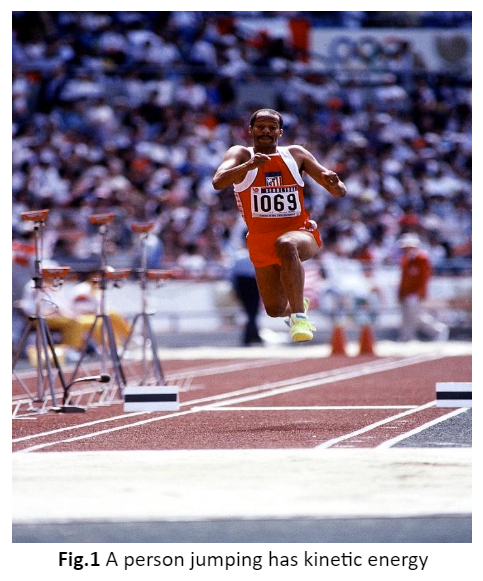Unit of Kinetic Energy
The S.I. unit used for the measurement of kinetic energy is joule or $kgm^2/s^2$.

The Formula for Kinetic Energy
The kinetic energy of the object depends on both its speed and motion. There is a mathematical relationship between the kinetic energy, mass, and speed of the object. kinetic energy can be determined by using the mentioned formula;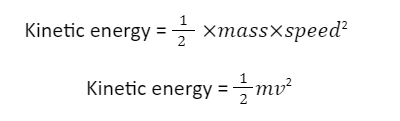Effect of Mass
The kinetic energy is affected by the mass of the object. It is directly proportional to the mass of the object, as it is shown in the above formula. When the mass of the object increases, the kinetic energy also increases. Similarly, kinetic energy decreases as the mass of the body decreases.

For example, a train has a very high amount of kinetic energy than a truck because the train has much more mass than the truck’s mass.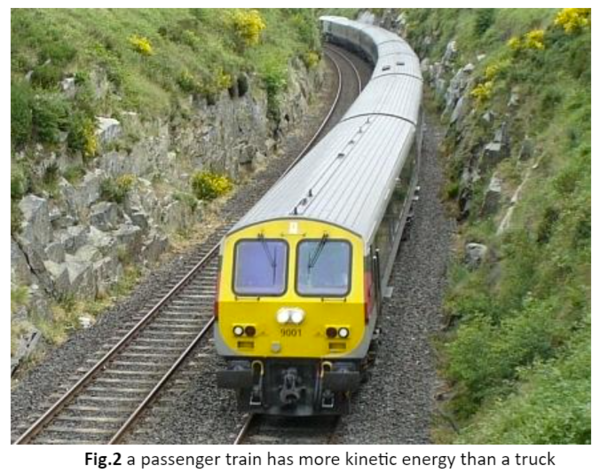Effect of Speed
The kinetic energy is also affected by the speed of the object, as it is directly proportional to the square speed of the object. If the speed of an object is doubled, it will increase the kinetic energy 4 times and vice versa.

For example, roller coasters are fun to ride. When the wagon of a roller coaster is at the top, it has zero kinetic energy because it is in the rest position. When it is allowed to move in free fall, its speed gradually increases. Then the gradual increase in the kinetic energy also takes place.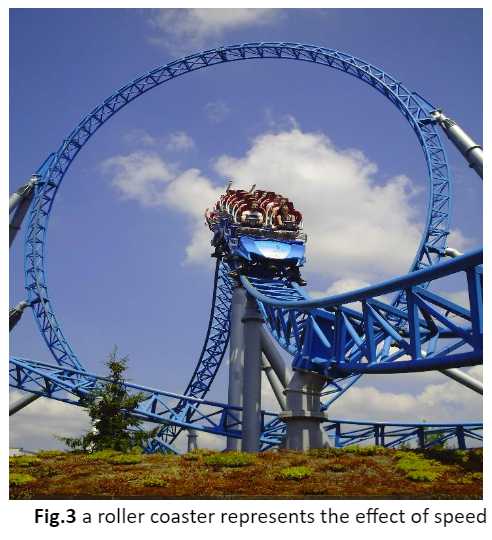Kinetic Energy Transformation
Kinetic energy can transfer between objects and can be transferred from one form of energy to another. For example, a bus moving down a hill is the best example to describe the transformation of kinetic energy. In the beginning, at the top of the hill, the bus has potential energy due to the height with almost zero kinetic energy. When the bus moves down the hill, the potential energy will start to decrease and there will be an increase in kinetic energy. There will come a point, where the value of both potential energy and kinetic energy will be the same. When the bus reaches the bottom of the hill, the kinetic energy will become maximum as far as it is moving with constant velocity. At this stage, the potential energy will become zero.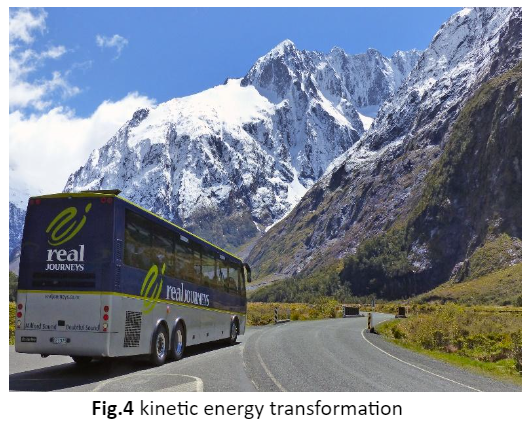Types of Kinetic Energy

The energy which is transferred with the help of waves or particles is known as radiant energy. The sunlight is a great example of radiant energy.

Thermal Energy
It is similar to radiant energy, but the difference is you experience this energy in the form of heat or warmth. We can observe this energy among the molecules and atoms in an object. For instance, boiling water on a stove is an example of thermal energy.

Sound Energy
This energy comes from vibration moving through something, e.g., when a force makes an object vibrate. The noise of the buzzing bee represents the sound energy.

Electrical Energy
It is associated with the flow of negatively charged electrons that produces electricity.

Summary
• Kinetic energy is the type of energy that an object possesses due to its motion.
• It is a scalar quantity that can be described by magnitude only.
• The kinetic energy depends on the mass as well as the speed of the body. A small change in one or both can affect the kinetic energy of the body.
• It is transferred between objects and can transfer from one form to another but is never destroyed. That proves the law of conservation of energy.

Related Worksheets: